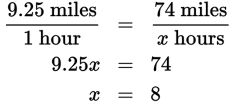# SAT Math Multiple Choice Question 522: Answer and Explanation

### Test Information

Question: 522

12. The human body has a very limited ability to store carbohydrates, which is why it is important for athletes to consume them during long training sessions or competitions. It is recommended that athletes consume approximately 3 calories per minute in situations like these. How many calories would an athlete biking a 74-mile race need to consume, assuming he bikes at an average speed of 9.25 miles per hour during the race?

• A. 480
• B. 1,440
• C. 1,665
• D. 2,053.5

Explanation:

B

Difficulty: Medium

Category: Problem Solving and Data Analysis / Rates, Ratios, Proportions, and Percentages

Strategic Advice: This is a question about rates, so pay careful attention to the units. As you read the question, decide how and when you will need to convert units.

Getting to the Answer: First, determine how long it will take the athlete to complete the race. Set up a proportion.The question asks for the total number of calories needed. The recommended rate of consumption is given in calories per minute, and you now know the number of hours that it will take the athlete to complete the race. So, you could convert the number of hours to minutes (8 × 60 minutes = 480 minutes) and then multiply this by 3 (the calorie per minute rate given) to find that the athlete should consume 480 × 3 = 1,440 calories. Or, you could also convert the given rate (3 calories per minute) to a per-hour rate (3 × 60 = 180 calories per hour) and then multiply this by the number of hours it will take the athlete to finish the race (180 × 8 = 1,440 calories).